# Identifying Discrete-Time State-Space Models (System Identification Toolkit)

LabVIEW 2013 System Identification Toolkit Help

Edition Date: June 2013

Part Number: 372458D-01

»View Product InfoDownload Help (Windows Only)

After physical modeling, you can describe the direct circuit (DC) servomotor by using the following differential equations: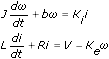Set Ki to be equal to Ke to transform these differential equations. If you want to design a digital state feedback controller, transform these differential equations to the following continuous-time state-space model: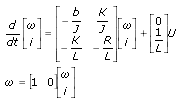You can transform the continuous-time state-space model to the following discrete-time state-space model: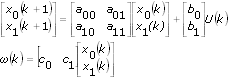where a00, a01, a10, a11, b0, b1, c0, c1 are unknown parameters. ω is the rotational speed of the shaft. U is the driving voltage.

You must estimate the values of the A, B, and C matrices to identify a state-space model. In this example, you must estimate the values of a00, a01, a10, a11, b0, b1, c0, c1 to identify the discrete-time state-space model.

## Using the SI Model Estimation Express VI

Complete the following steps to identify the discrete-time state-space model by using the SI Model Estimation Express VI.

1. Add the SI Model Estimation Express VI to the block diagram of the VI you created.

The Configure SI Model Estimation dialog box appears.
2. Select the State-Space button in the Select Model Type section to identify state-space models. The Model Diagram section displays a mathematical equation for the selected model type and a diagram of the model.
3. Select the SISO button in the Select System Dims section to identify single-input single-output (SISO) dynamic models.
4. Select the Waveform button in the Select Data Type section to identify dynamic models by using waveform input signals.
5. Enter 2 in the Number of states text box because this model contains two states: rotational speed ω and armature current i.
6. Click the OK button to close the configuration dialog box and return to the block diagram.
7. Wire the stimulus signal and response signal outputs of the SI Data Samples VI to the corresponding inputs of the SI Model Estimation Express VI.
8. Add the SI Draw Model VI to the block diagram.

9. Wire the system model out output of the SI Model Estimation Express VI to the system model input of the SI Draw Model VI.
10. Create an indicator for the model output of the SI Draw Model VI. The following figure shows the block diagram.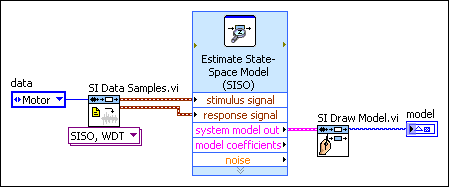11. Wire all error in and error out terminals on the block diagram.
12. Run this VI to identify the dynamic system model.

## Using the SI Estimate State-Space Model VI

Complete the following steps to identify the discrete-time state-space model by using the SI Estimate State-Space Model VI.

1. Add the SI Estimate State-Space Model VI to the block diagram of the VI you created.

2. Wire the stimulus signal and response signal outputs of the SI Data Samples VI to the corresponding inputs of the SI Estimate State-Space Model VI.
3. Create a control for the structure selector input of the SI Estimate State-Space Model VI.
4. Set all the Boolean controls of the structure selector input to FALSE because you need to estimate the A, B, and C matrices only.
5. Create a control for the number of states input of the SI Estimate State-Space Model VI.
6. Set the number of states input to 2 because the continuous-time state-space model contains two states: rotational speed ω and armature current i.
7. (Optional) Switch to the front panel, right-click all controls, and select Data Operations»Make Current Value Default from the shortcut menu. LabVIEW uses the current values as the default values for these controls.
8. Add the SI Draw Model VI to the block diagram.

9. Wire the system model out output of the SI Estimate State-Space Model VI to the system model input of the SI Draw Model VI.
10. Create an indicator for the model output of the SI Draw Model VI. The following figure shows the block diagram.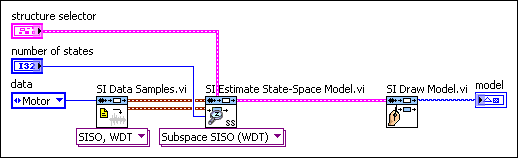11. Wire all error in and error out terminals on the block diagram.
12. Run this VI to identify the system model.

## Verifying Resulting Dynamic System Models

If you use the SI Data Samples VI to generate the input and output data in Part 2, LabVIEW returns the following system model: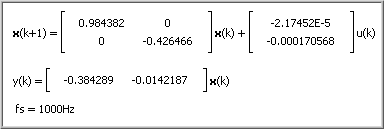where a00= 0.984382. a01= 0. a10= 0. a11= –0.426466. b0= –2.17452E–5. b1= –0.000170568. c0= –0.384289. c1= –0.0142187.